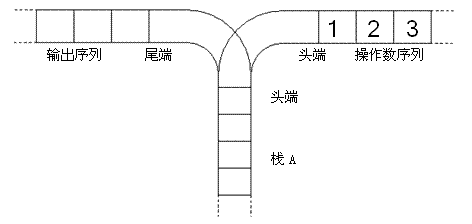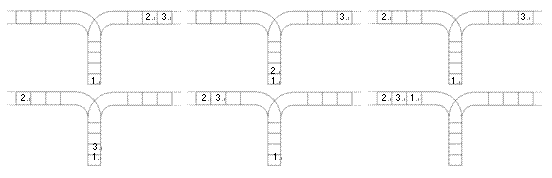# P1044 栈(内含推导过程)

，其中一个是 1-k-1，序列个数为k-1，另外一个是k+1-n，序列个数是n-k。此时，如果我们把k视为一个序数，那么根据乘法原理，f(n)的问题等价于--序列个数为k-1的出栈序列种数*序列个数为 n-k的出栈序列种数，即选择k这个序数的

f（n）= f(k-1)*f(n-k)，而k可以选择1-n，再根据加法原理,将k取不同值的序列种数相加，

f(n) =f(0)*f(n-1) + f(1)*f(n-2)+...+f(n-1)*f(0)

## 题目描述1. 将一个数，从操作数序列的头端移到栈的头端（对应数据结构栈的 push 操作）
2. 将一个数，从栈的头端移到输出序列的尾端（对应数据结构栈的 pop 操作）（原始状态如上图所示）

## 输入输出样例

3


5
#include<iostream>
#define maxn 20
typedef long long LL;
using namespace std;
LL f[maxn];
int main(){
int n;
cin>>n;
f = 1;
f = 1;
for(int i=2;i<=n;i++)
for(int j=0;j<i;j++)
f[i] +=f[j]*f[i-1-j];
cout<<f[n];
return 0;
}©️2019 CSDN 皮肤主题: 大白 设计师: CSDN官方博客